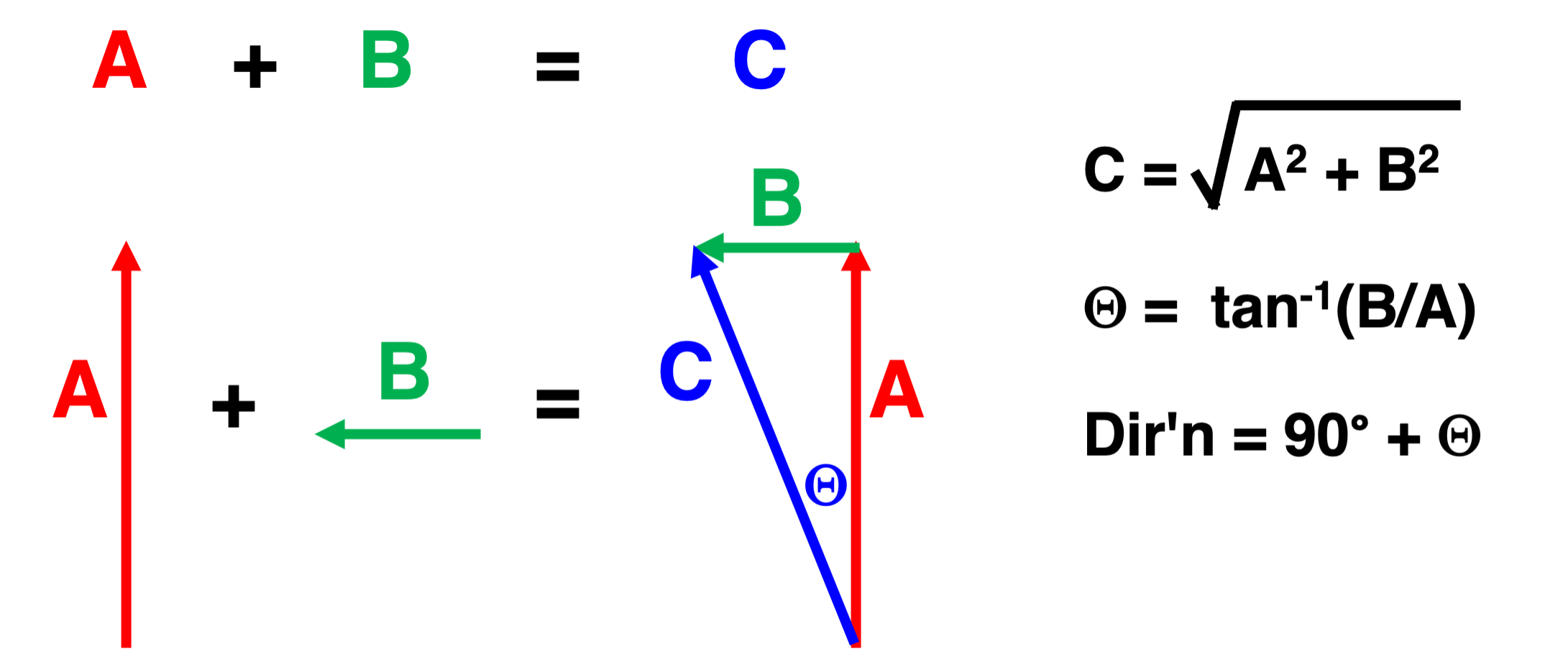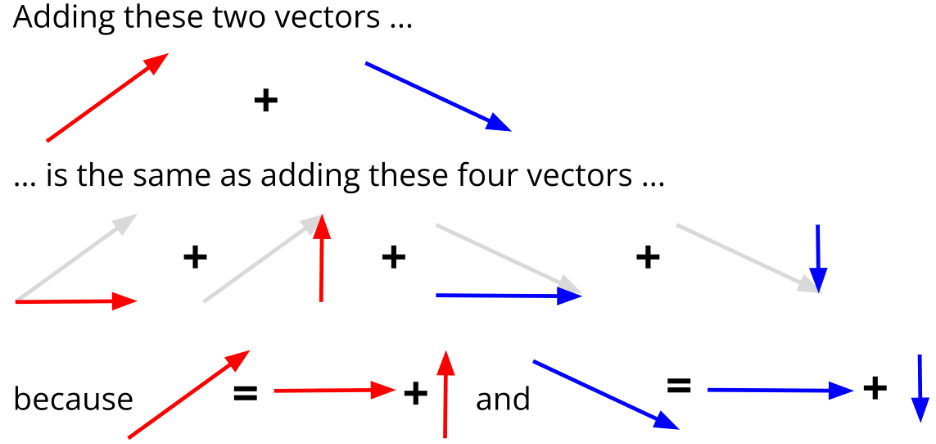# Electricity: Static Electricity

## Static Electricity: Problem Set Overview

There are 17 ready-to-use problem sets on the topic of Static Electricity. The problems targets your ability to determine quantities such as the quantity of charge, separation distance between charges, electric force, electric field strength, and resultant forces and field strengths from verbal descriptions and diagrams of physical situations pertaining to charged objects. Problems range in difficulty from the very easy and straight-forward to the very difficult and complex.

## Relating the Quantity of Charge to Numbers of Protons and Electrons

Atoms are the building blocks of all objects. These atoms possess protons, neutrons and electrons. While neutrons are electrically neutral, the protons and electrons possess electrical charge. The proton and electron have a predictable amount of charge, with the proton being assigned a positive type of charge and the electron a negative type. The charge on an electron has a well-accepted, experimentally-determined value of -1.6 x 10-19 C (where the negative simply indicates the type of charge). Protons have an equal amount of charge and an opposite type; thus, the charge of a proton is +1.6 x 10-19 C. Objects consisting of atoms containing protons and electrons can have an overall charge on them if there is an imbalance of protons and electrons. An object with more protons than electrons will be charged positively and an object with more electrons than protons will be charged negatively. The magnitude of the quantity of charge on an object is simply the difference between the number of protons and electrons multiplied by 1.6 x 10-19 C.

## Coulomb's Law of Electric Force

A charged object can exert an attractive or repulsive force on other charged objects in its vicinity. The amount of force follows a rather predictable pattern which is dependent upon the amount of charge present on each of the two objects and the distance of separation between the objects' centers. Coulomb's law of electric force expresses the relationship in the form of the following equation:

Felect = k•Q1•Q2/d2

where Felect represents the magnitude of the electric force (in Newtons), Q1 and Q2 represent the quantity of charge (in Coulombs) on objects 1 and 2, and d represents the separation distance between the objects' centers (in meters). The symbol k represents a constant of proportionality known as Coulomb's constant and has the value of 8.99 x 109 N•m2/C2.

## Electric Field

A charged object can exert an electric influence upon other objects from which they are spatially separated. This action-at-a-distance phenomenon is sometimes explained by saying the charged object establishes an electric field in the space surrounding it. Other objects which enter the field interact with the field and experience the influence of the field. The strength of the electric field can be tested by measuring the force exerted on a test charge. Of course, the more charge on the test charge, the more force which would be experienced by it. While the force experienced by the test charge is proportional to the amount of charge on the test charge, the ratio of force to charge would be the same regardless of the amount of charge on the test charge. By definition, the electric field strength (E) at a given location about a source charge is simply the ratio of the force experienced (F) by a test charge to the quantity of charge on the test charge (qtest).

E = F / qtest

The electric field strength as created by a source charge (Q) varies with location. In accord with Coulomb's law, the force on a test charge is greatest when closest to the source charge and less when further away. Substitution of the expression for force into the above equation and subsequent algebraic simplification yields a second equation for electric field (E) which expresses its strength in terms of the variables which effect it. The equation is

E = k • Q / d2

where k is Coulombs constant of 8.99.0 x 109 N•m2/C2, Q is the quantity of charge on the source creating the field and d is the distance from the center of the source.

## Direction of Force and Field Vectors

Many problems in this problem set will demand that you understand the directional nature of electric force and electric field. Electric forces between objects can be attractive or repulsive. Objects charged with an opposite type of charge will be attracted to each other and objects charged with the same type of charge will be repelled by each other. These attractive and repulsive interactions describe the direction of the forces exerted upon any object. In some instances involving configurations of three or more charges lying along a line, an object will experience two or more forces in the same or different directions. In such instances, the interest is usually in knowing what the net electric force is. Finding the net electric force involves determining the magnitude and direction of the individual forces and then adding them up as vectors to determine the net force. When adding electric forces, the direction must be considered. A 10 unit force to the left and a 25 unit force to the right add up to a 15 unit force to the right. Such reasoning about direction will be critical to analyzing situations where two or more forces are present.

Electric field is also a vector quantity that has a directional nature associated with it. By convention, the direction of the electric field vector at any location surrounding a source charge is in the direction that a positive test charge would be pushed or pulled if placed at that location. Even if a negative charge is used to measure the strength of a source charge's field, the convention for direction is based upon the direction of force on a positive test charge.

## Adding Vectors - SOH CAH TOA and Pythagorean Theorem

Electric field and electric force are vector quantities that have a direction. In situations in which there are two or more force or field vectors present, it is often desired to know what the net electric force or field is. Finding the net value from knowledge of individual values requires that vectors be added together in head-to-tail fashion. If the vectors being added are at right angles to each other, then the Pythagorean theorem can be used to determine the resultant or net value; a trigonometric function can be used to determine an angle and subsequently a direction.If the vectors being added are not at right angles to each other, then the usual procedure of adding them involves using a trigonometric function to resolve each vector into x- and y-components. The components are then added together to determine the sum of all x- and y-components. These sum values can then be added together in a right triangle to determine the net or resultant vector. And as usual, a trigonometric function can be used to determine an angle and subsequently a direction of the net or resultant vector. The graphic below depicts by means of diagrams how the components of a vector can be added together to determine the resultant of two vectors that are not perpendicular to one another.The more difficult problems in this unit involve analyzing situations in which force and field vectors are not perpendicular to one another. Our video titled Solving DIfficult Electric Field Problems will be of much help.

## Comparing Gravitational and Electrical Forces

Gravitational forces and electrical forces are often compared to each other. Both force types are fundamental forces which act over a distance of separation. Gravitational forces are based on masses attracting and follow the universal gravitation equation.

Fgrav = G • m1 • m2 / d2

where m1 and m2 are the masses of the attracting objects (in kg), d is the separation distance as measured from object center to object center (in meters) and G is a proportionality constant with a value of 6.673 x 10-11 N•m2/kg2.

Electrical forces are based on charged objects attracting or repelling and follow the Coulomb's law equation (as discussed above). Some of the problems on this set will involve comparisons of the magnitude of the electric force to the magnitude of the gravitational force. The simultaneous use of both equations will be necessary in the solution of such problems.

## Habits of an Effective Problem-Solver

An effective problem solver by habit approaches a physics problem in a manner that reflects a collection of disciplined habits. While not every effective problem solver employs the same approach, they all have habits which they share in common. These habits are described briefly here. An effective problem-solver...

• ...reads the problem carefully and develops a mental picture of the physical situation. If needed, they sketch a simple diagram of the physical situation to help visualize it.
• ...identifies the known and unknown quantities and records them in an organized manner, often times recording them on the diagram itself. They equate given values to the symbols used to represent the corresponding quantity (e.g., Q1 = 2.4 μC; Q2 = 3.8 μC; d = 1.8 m; Felect = ???).
• ...plots a strategy for solving for the unknown quantity; the strategy will typically center around the use of physics equations and be heavily dependent upon an understanding of physics principles.
• ...identifies the appropriate formula(s) to use, often times writing them down. Where needed, they perform the needed conversion of quantities into the proper unit.
• ...performs substitutions and algebraic manipulations in order to solve for the unknown quantity.